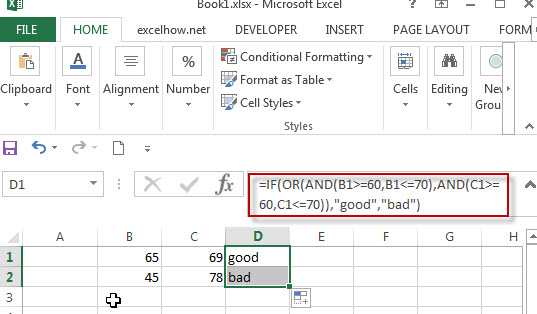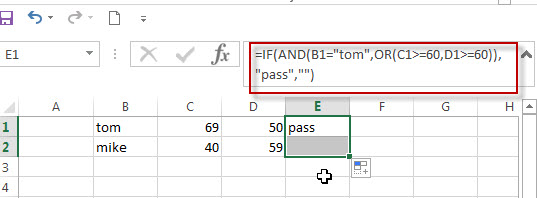# Excel IF formula with AND & OR logical functions

If you want to test the result of cells based on several sets of multiple test conditions, you can use the IF function with the AND and OR functions at a time.

Assuming that you have the following test logic to check the exam scores:

Logic test1: 60<=B1<=70

Logic test2: 60<=C1<=70

If any of the above conditions are met, then mark the result as “good”, otherwise, mark the result as “bad”. We need to use two AND functions to reflect the above two logical test and enclose them in the OR function as one logical test in the Excel IF formula. Let’s write down the below IF formula with AND & OR logical functions:

`=IF(OR(AND(B1>=60,B1<=70),AND(C1>=60,C1<=70)),"good","bad") `Let’s see another IF formula with AND and OR functions as follows:

`=IF(AND(B1="tom",OR(C1>=60,D1>=60)),"pass","")`

When B1 is equal to “tom”, and either C1 is greater than or equal to “60” or D1 is greater than or equal to 60, the formula returns “pass”. Otherwise, the formula will return an empty string.If we just want to check the cells where the value in column B is “tom” AND the score in column C is greater than or equal to 60. If you want to return TRUE, you can use the below logical test statement with the AND function:

`AND(B1="tom",C1>=60)`

Next, we can extend the above statement, we want to check the cells if the values in column B is “tom” AND the scores in column C or column D is greater than or equal to 60. Then we can nest the OR function in the above AND function like below:

`AND(B1="tom",OR(C1>=60,D1>=60))`

### Related Formulas

• Excel IF formula with equal to logical operator
The “Equal to” logical operator can be used to compare the below data types, such as: text string, numbers, dates, Booleans.  This section will guide you how to use “equal to” logical operator in excel IF formula with text string value and dates value…
• Excel IF formula with OR logical function
If you want to check if one of several conditions is met in your excel workbook, if the test is TRUE, then you can take certain action. You can use the IF function combining with OR function to construct a condition statement…
• Excel IF formula with AND logical function
You can use the IF function combining with AND function to construct a condition statement. If the test is FALSE, then take another action…

### Related Functions

• Excel AND function
The Excel AND function returns TRUE if all of arguments are TRUE, and it returns FALSE if any of arguments are FALSE.The syntax of the AND function is as below:= AND (condition1,[condition2],…) …
• Excel OR function
The Excel OR function used to test multiple conditions and returns TRUE if any of the conditions are TRUE. Otherwise, it will return FALSE. The syntax of the OR function is as below:=OR(logical1, [logical2], …)…
• Excel IF function
The Excel IF function perform a logical test to return one value if the condition is TRUE and return another value if the condition is FALSE….
• Excel nested if function
The nested IF function is formed by multiple if statements within one Excel if function. This excel nested if statement makes it possible for a single formula to take multiple actions…